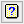1. Home
2. Microarray One Channel ANOVA Store Options

# Microarray One Channel ANOVA Store Options

Use this to save results from a one channel analysis of variance. These results are saved when an analysis is run and must be specified before running an analysis.

1. After selecting the appropriate boxes, type names for the data structures into the corresponding In fields.## Save

The table below indicates the type of structures formed for each item.

 Probe/Gene IDs Text The list of probes/gene identifiers to index the results. Means Pointer The means for each treatment term will be saved in unnamed matrices pointed to by this pointer. The treatment levels will be in the columns of the matrix and there will be one row for each probe. Variance-covariance matrix Pointer The symmetrical variance-covariance matrix of the means will be saved in unnamed matrices pointed to by this pointer. The items of the n x (n-1)/2 variance-covariance matrix will be in the columns of the matrix and there will be one row for each probe. Effects Pointer The effects for each treatment term will be saved in unnamed matrices pointed to by this pointer. The treatment levels will be in the columns of the matrix and there will be one row for each probe. Unit variance of effects Pointer The unit variance of the stratum that each treatment term is in will be saved in unnamed variates pointed to by this pointer. The variates contains the values for each probe. Standard errors of effects Pointer The standard errors of the effects for each treatment term will be saved in unnamed matrices pointed to by this pointer. The treatment levels will be in the columns of the matrix and there will be one row for each probe. Treatment degrees of freedom Pointer The degrees of freedom for each treatment will be saved in unnamed variates pointed to by this pointer. These are stored in unnamed variates for each treatment term which are pointed to by this pointer. Treatment sums of squares Pointer The sums of squares for each treatment will be saved in unnamed variates pointed to by this pointer. These are stored in unnamed variates for each treatment term which are pointed to by this pointer. Treatment mean square Pointer The mean of square (i.e. the sums of squares divided by its degrees of freedom) for each treatment will be saved in unnamed variates pointed to by this pointer. Residual degrees of freedom Pointer The residual degrees of freedom in the stratum each treatment occurs in each probe. These are stored in unnamed variates for each treatment term which are pointed to by this pointer. Residual sums of squares Pointer The residual sums of squares in the stratum each treatment occurs in from the ANOVA for each probe. These are stored in unnamed variates for each treatment term which are pointed to by this pointer. Residual mean square Pointer The residual mean square in the stratum each treatment occurs in each probe. These are stored in unnamed variates for each treatment term which are pointed to by this pointer. Treatment variance/F ratio Pointer The variance or F ratio (i.e. the treatment mean squares divided by the residual mean square) for each treatment will be saved in unnamed variates pointed to by this pointer. Treatment F probability Pointer The probability of a F statistic being more extreme than the observed the F ratio for each treatment will be saved in unnamed variates pointed to by this pointer. Contrast estimates Pointer The contrast estimates will be saved in unnamed matrices pointed to by this pointer. The contrasts will be in the columns of the matrix and there will be one row for each probe. There will be one matrix for each treatment term which has contrasts defined for it. Where not all the degrees of freedom of the treatment term are used by the contrasts, extra terms giving the deviations for each treatment level will also be saved. Standard errors of contrasts Pointer The contrast standard errors will be saved in unnamed matrices pointed to by this pointer. The standard errors will be in the columns of the matrix and there will be one row for each probe. Contrast t-values Pointer The contrast t-values (the contrasts divided by their standard errors) will be saved in unnamed matrices pointed to by this pointer. The T values will be in the columns of the matrix and there will be one row for each probe. Probabilities of contrasts Pointer The two sided probabilities of contrast t-values being more extreme that the observed t-values will be saved in unnamed matrices pointed to by this pointer.

Select this to display the results in a new spreadsheet window.

## Save results as variates in a single spreadsheet

The saved results will be displayed in a single spreadsheet where matrices are converted to variate columns. The variate columns that are derived from matrices will be renamed with a standard name and includes a column description that specifies the treatment term and level/contrast it originates from.

If this is not selected, then the matrices will be loaded into a number of separate spreadsheets, one for each treatment term by result type (effects, SEs etc).

## Action IconsClear Clear all fields and list boxes.Help Open the Help topic for this dialog.